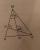# 30-60-90

The longer leg of a 30°-60°-90° triangle measures 5. What is the length of the shorter leg?

Result

x =  2.5

#### Solution:Try calculation via our triangle calculator.

Leave us a comment of example and its solution (i.e. if it is still somewhat unclear...):

Showing 0 comments:Be the first to comment!#### To solve this example are needed these knowledge from mathematics:

See also our right triangle calculator. See also our trigonometric triangle calculator.

## Next similar examples:

1. Double ladderThe double ladder shoulders should be 3 meters long. What height will the upper top of the ladder reach if the lower ends are 1.8 meters apart?
2. Cable carCable car rises at an angle 45° and connects the upper and lower station with an altitude difference of 744 m. How long is "endless" tow rope?
3. Right triangleLadder 16 feet reaches up 14 feet on a house wall. The 90-degree angle at the base of the house and wall. What are the other two angles or the length of the leg of the yard?
4. ReflectorCircular reflector throws light cone with a vertex angle 49° and is on 33 m height tower. The axis of the light beam has with the axis of the tower angle 30°. What is the maximum length of the illuminated horizontal plane?
5. Cable car 2Cable car rises at an angle 41° and connects the upper and lower station with an altitude difference of 1175 m. How long is the track of cable car?
6. Double ladderThe double ladder is 8.5m long. It is built so that its lower ends are 3.5 meters apart. How high does the upper end of the ladder reach?
7. Equilateral triangleHow long should be the minimum radius of the circular plate to be cut equilateral triangle with side 19 cm from it?
8. Obtuse angleThe line OH is the height of the triangle DOM, line MN is the bisector of angle DMO. obtuse angle between the lines MN and OH is four times larger than the angle DMN. What size is the angle DMO? (see attached image)
9. Hexagon 5The distance of parallel sides of regular hexagonal is 61 cm. Calculate the length of the radius of the circle described to this hexagon.
10. Right triangleCalculate the length of the remaining two sides and the angles in the rectangular triangle ABC if a = 10 cm, angle alpha = 18°40'.
11. Height 2Calculate the height of the equilateral triangle with side 38.
12. An angleAn angle x is opposite side AB which is 10, and side AC is 15 which is hypotenuse side in triangle ABC. Calculate angle x.
13. ClimbFor horizontal distance 4.9 km road rise by 6.1 m. Calculate the road pitch in ‰ (permille, parts per thousand).
14. Center traverseIt is true that the middle traverse bisects the triangle?
15. SinesIn ▵ ABC, if sin(α)=0.5 and sin(β)=0.6 calculate sin(γ)
16. Find the 9Find the missing angle in the triangle and then name triangle. Angles are: 95, 2x+15, x+3
17. SlopeFind the slope of the line: x=t and y=1+t.